AP State Board Syllabus AP SSC 10th Class Maths Textbook Solutions Chapter 1 Real Numbers Ex 1.2 Textbook Questions and Answers.

## AP State Syllabus SSC 10th Class Maths Solutions 1st Lesson Real Numbers Exercise 1.2

### 10th Class Maths 1st Lesson Real Numbers Ex 1.2 Textbook Questions and Answers

Question 1.
Express each of the following numbers as a product of its prime factors.
i) 140
ii) 156
iii) 3825
iv) 5005
v) 7429
i) 140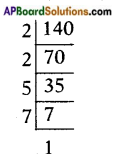∴ 140 = 2 × 2 × 5 × 7 = 22 × 5 × 7ii) 156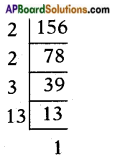∴ 156 = 2 × 2 × 3 × 13 = 22 × 3 × 13

iii) 3825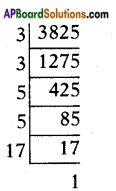∴ 3825 = 3 × 3 × 5 × 5 × 17 = 32 × 52 × 17

iv) 5005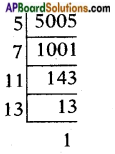∴ 5005 = 5 × 7 × 11 × 13

v) 7429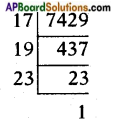∴ 7429 = 17 × 19 × 23Question 2.
Find the L.C.M and H.C.F of the following integers by the prime factorization method.
i) 12, 15 and 21
ii) 17, 23 and 29
iii) 8, 9 and 25
iv) 72 and 108
v) 306 and 657
i) 12, 15 and 21
12 = 2 × 2 × 3 = 22 × 3
15 = 3 × 5
21 = 3 × 7
L.C.M = 22 × 3 × 5 × 7 = 420
H.C.F = 3

ii) 17, 23 and 29
The given numbers 17, 23 and 29 are all primes.
L.C.M = their product
= 17 × 23 × 29 = 11339
∴ H.C.F = 1

iii) 8, 9 and 25
8 = 2 × 2 × 2 = 23
9 = 3 × 3 = 32
25 = 5 × 5 = 52
L.C.M = 23 × 32 × 52 = 1800
(or)
8, 9 and 25 are relatively prime, therefore L.C.M is equal to their product,
(i.e.,) L.C.M = 8 × 9 × 25 = 1800
H.C.F = 1

iv) 72 and 108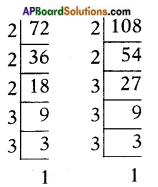72 = 23 × 32
108 = 22 × 33
L.C.M = 23 × 33 = 8 × 27 = 216
H.C.F = 22 × 32 = 4 × 9 = 36v) 306 and 657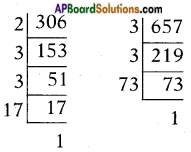306 = 2 × 32 × 17
657 = 32 × 73
L.C.M = 2 × 32 × 17 × 73 = 22338
H.C.F = 32 = 9

Question 3.
Check whether 6n can end with the digit ‘0’ for any natural number n.
Given number = 6n = (2 × 3)n
The prime factors here are 2 and 3 only.
To be end with 0; 6n should have a prime factor 5 and also 2.
So, 6n can’t end with zero.

Question 4.
Explain why 7 × 11 × 13 + 13 and 7 × 6 × 5 × 4 × 3 × 2 × 1 + 5 are composite numbers.
Given numbers are 7 × 11 × 13
7 × 6 × 5 × 4 × 3 × 2 × 1 + 5
⇒ 13(7 × 11 + 1) and
5(7 × 6 × 4 × 3 × 2 × 1 + 1)
⇒ 13 K and 5 L, where K = 78 and L = 7 × 6 × 4 × 3 × 2 × 1 + 1 = 1009
As the given numbers can be written as product of two numbers, they are composite.

Question 5.
How will you show that (17 × 11 × 2) + (17 × 11 × 5) is a composite number? Explain.
(17 × 11 × 2) + (17 × 11 × 5)
= (17 × 11) (2 + 5)
= (17 × 11) (7)
= 187 × 7
Now the given expression is written as a product of two integers and hence it is a composite number.Question 6.
What is the last digit of 6100?